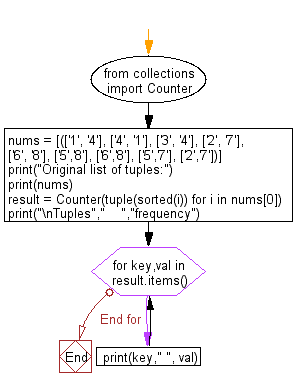﻿ Python: Frequency of the tuples in a given list - w3resource# Python: Frequency of the tuples in a given list

## Python Collections: Exercise-23 with Solution

Write a Python program to get the frequency of the tuples in a given list.

Sample Solution:

Python Code:

``````from collections import Counter
nums = [(['1', '4'], ['4', '1'], ['3', '4'], ['2', '7'], ['6', '8'], ['5','8'], ['6','8'], ['5','7'], ['2','7'])]
print("Original list of tuples:")
print(nums)
result = Counter(tuple(sorted(i)) for i in nums)
print("\nTuples","    ","frequency")
for key,val in result.items():
print(key," ", val)
```
```

Sample Output:

```Original list of tuples:
[(['1', '4'], ['4', '1'], ['3', '4'], ['2', '7'], ['6', '8'], ['5', '8'], ['6', '8'], ['5', '7'], ['2', '7'])]

Tuples      frequency
('1', '4')   2
('3', '4')   1
('2', '7')   2
('6', '8')   2
('5', '8')   1
('5', '7')   1
```

Flowchart:## Visualize Python code execution:

The following tool visualize what the computer is doing step-by-step as it executes the said program:

Python Code Editor:

Have another way to solve this solution? Contribute your code (and comments) through Disqus.

What is the difficulty level of this exercise?

Test your Programming skills with w3resource's quiz.

﻿

## Python: Tips of the Day

The Zip() Function:

```>>> students = ('John', 'Mary', 'Mike')
>>> ages = (15, 17, 16)
>>> scores = (90, 88, 82, 17, 14)
>>> for student, age, score in zip(students, ages, scores):
...     print(f'{student}, age: {age}, score: {score}')
...
John, age: 15, score: 90
Mary, age: 17, score: 88
Mike, age: 16, score: 82
>>> zipped = zip(students, ages, scores)
>>> a, b, c = zip(*zipped)
>>> print(b)
(15, 17, 16)
```